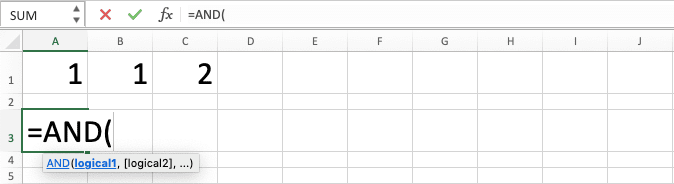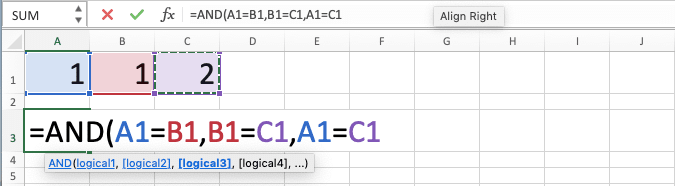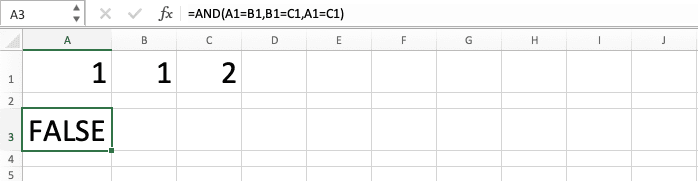How to Use AND Function in Excel: Usabilities, Examples, and Writing Steps - Compute Expert

# How to Use AND Function in Excel: Usabilities, Examples, and Writing Steps

Home >> Excel Tutorials from Compute Expert >> Excel Formulas List >> How to Use AND Function in Excel: Usabilities, Examples, and Writing Steps

In this tutorial, you will learn completely about AND function in excel.

When we need to process multiple logic conditions to get its combined logic value, it is troublesome to do it manually. AND can help us to process them fast, as long as its unique processing is what we need.

Disclaimer: This post may contain affiliate links from which we earn commission from qualifying purchases/actions at no additional cost for you. Learn more

## AND Function in Excel

We can use AND to get a combined value of more than one logic condition. It will produce TRUE if all those logic conditions are true/correct and FALSE if not.

## AND Result

We get a TRUE/FALSE logic value from AND, depending on the true/false of all of its logic condition inputs.

## Excel Version from Which We Can Use AND Formula in Excel

We can use AND since excel version 2003.

## The Way to Write It and Its Inputs

Here is the general writing form of an AND formula in excel.

= AND ( logic_condition_1 , logic_condition_2 , … )

In AND, we input all the logic conditions from where we want to get the combined logic value. We separate those logic condition inputs using comma signs ( , ).

## Example of Its Usage and Result

The following is the implementation and result example of AND in excel.In the example, we try to know the pass/fail results for each row of test scores. If all of them are above 70, then it passes. On the other hand, if one of them isn’t above 70, then it fails.

To get the pass/fail results fast for all the test score rows, we use the AND formula.

As you can see in the example, we input all the test score logic conditions per row in our AND. As the requirement is above 70 for all the test scores, we create the logic condition inputs like that (test_score>70).

We separate all the AND logic condition inputs using comma signs as we discuss in the previous tutorial part.

Write the AND correctly and we will get the combined result of the logic conditions we input fast!

## Writing Steps

Next, we will see how to write the AND formula in excel from the beginning until the end. The writing steps are pretty simple as you can see in the following.

1. Type an equal sign ( = ) in the cell where you want to put the AND result in2. Type AND (can be with large and small letters) and an open bracket sign after =3. Input all the logic conditions from which you want to get the combined logic value. Separate them with comma signs ( , )4. Type a close bracket sign after you have inputted all your logic conditions5. Press Enter
6. The process is done!## AND for IF with Multiple Logic Conditions: IF AND

As the result of AND is a TRUE/FALSE logic value, we usually don’t use it on its own. Instead, we use it as a logic condition input in an IF formula.

If you have known IF, then you know that it needs a logic condition input that produces a logic value. That logic value will determine which result that IF is going to produce.

However, in its normal writing, IF can only accept one logic condition. If you want to get a combined value of multiple logic conditions, then you need to use AND in the IF (or OR if you need different processing for the logic conditions combination).

The way to write IF with AND in excel is usually as follows.

= IF ( AND ( logic_condition_1 , logic_condition_2 , … ) , result_if_true , result_if_false )

As we discuss earlier, we write AND as the logic condition input in IF. AND will help to combine multiple logic conditions to determine the result of our IF.

If all the logic conditions are TRUE, then IF will produce its TRUE result. Otherwise, IF will produce its FALSE result.

To understand more about the IF AND implementation in excel, see the screenshot below.We go back to the test scores example earlier and add IF to determine the result there. If all the test scores are above 70, then we want the “pass” mark. Otherwise, we want the “fail” mark.

To give those marks for all the test score rows, we input the AND formula in our IF. The AND contains all the logic conditions for each test score in the row. Each of them must be above 70.

We input the “pass” mark as the TRUE result of the IF and the “fail” mark as its FALSE result.

Write the IF AND like that and we will get the result we need!

## AND Alternative: Multiply Your Logic Conditions

If you don’t want to write an AND to combine your logic conditions, then you can use this alternative method. It utilizes the fact that TRUE is also recognized as 1 and FALSE as 0 in excel.

Can you infer what we will do based on that? We can multiply the logic conditions that we have to imitate the AND process because of that!

In writing, we can illustrate this alternative method generally as follows.

= ( logic_condition_1 ) * ( logic_condition_2 ) * …

The logic conditions that we write will produce TRUE or FALSE logic values. As TRUE is 1 and FALSE is 0 in excel, multiplying those logic values will give us a result like AND.

If all of them are TRUE (or 1), then the multiplication will produce 1 (or TRUE). On the other hand, if even just one of them is FALSE (0), then the multiplication will produce 0 (or FALSE). That is because anything that we multiply with 0 will result in 0!

The result is just like what we get from AND, right? We add brackets to envelop each logic condition to make them run first before the multiplication. This is to make sure that the process will run as intended.

As a note, if you run this writing on its own, then you will get a number (1 or 0, depending on whether the result is TRUE or FALSE). However, if you run it in an IF, then it will work just like a standard IF AND!

To illustrate the method implementation, take a look at the example below.We use the IF AND example from before to illustrate its similarity with if we use the logic conditions multiplication. As you can see, the results from both methods are just the same!

Here, we multiply the logic conditions using the writing pattern explained before. All of the test scores in each row are compared to the passing grade before we put them in brackets. After that, we multiply them using the star symbol ( * ).

We write the IF normally, only changing the AND to the logic conditions multiplication. As a result, we also get the “pass” or “fail” marks correctly from our formulas’ writings.

## Exercise

After you have learned how to use AND function in excel completely, now let’s do an exercise. This is so you can enhance your practical knowledge about the AND implementation in excel!

### Questions

1. How is the comparison of variable A indicators to the value in the first row on the corresponding column? Give ‘OK’ if all indicators exceed their value comparison and ‘X’ if not!
2. How is the comparison of variable B indicators to the value in the first row on the corresponding column? Give ‘OK’ if all indicators exceed their value comparison and ‘X’ if not!
3. How is the comparison of variable C indicators to the value in the first row on the corresponding column? Give ‘OK’ if all indicators exceed their value comparison and ‘X’ if not!

• We can input TRUE or FALSE logic values directly to our AND although it is rare to do it that way
• If we input text values or empty cells to an AND, it will ignore them
• AND isn’t case sensitive

Other tutorials you should learn:

Get updated excel info from Compute Expert by registering your email. It's free!